RF - Dielectric (Material/Constant)                                        Home : www.sharetechnote.com

One of the most important material in modern RF design would be 'Dielectric (material)' and most important concept (property) would be 'Dielectric Constant'. But it seems to be very tricky to explain on what 'Dielectric' practically means and why it became so important in most of RF component design.

I will try to explain on 'Dielectric' and related concepts in various different aspects. (But not at once, it would take a couple of weeks for me to complete this page... this is just beginning..)

 Conductor No Electric Field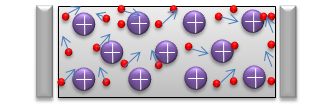There are a lot of free electrons moving around randomly. Electric Field Applied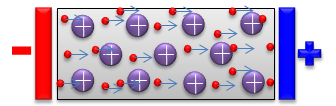There are a lot of free electrons moving towards the positive charge and this movement of free electrons creates 'current' Dielectric No Electric Field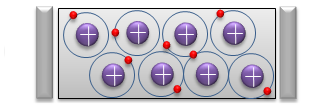Most of electrons are stay within the atom and stick around the positive nuclear. Electric Field Applied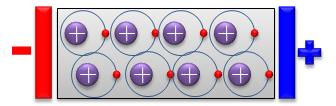Electrons are attracted towards to positive electrode and positive nuclear are attracted toward negative electrode,but they does not go out of a certain boundary. This alignment create a dipole (di-electric)

Dielectric Constant

We briefly thought about intuitive meaning of 'Dielectric'. Now let's think of 'quantitive' aspect of 'Dielectric property'. We call the quantative aspect of 'Dielectric property' as Dielectric Constant. As shown below, Dielectric Constant is the relative permittivity with reference to the permitivity of Vacumm. (Unfortunately we have another terminology called 'permitivity'). Does it remind you of the nightmare in getting lost in the mingle of words when you try to figure out the meaning of a word in the dictionary. The dictionary explains on the word using other words which you don't understand... and if you look into that unknown words in the dictionary you would find another word that you don't understand.. and then you never get out of the loop. As far as I know, there is no clear solution for this kind of situation unfortunately.. you have to live with this -:)

One tip is .. just get the meaning out of the basic mathematical definition and try to apply it in more practical situation as much as possible.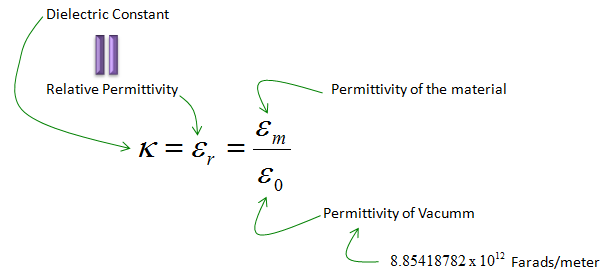If we define the dielectric constant using the term 'Permitivity', it may not sound intuitive to many readers, but it may sound more familiar if we define it as follows. It defines Dielectric Constant using Capacitance. Suppose that you have two capacitance with exactly same dimension and same material for conductor plates. The only difference is the material filled in between the two conductor plates which makes the capacitor. In one capacitor, the space between the two plates is filled with vacume. In the other capacitor, the space between the two plates is filled with a dielectric material. And then you measure the capacitance of the two capacitors and you will get different values. If you take the ratio of the two measured capacitance (capacitance of the one with Vacume as denominator), it becomes the dielectric Constance of the material.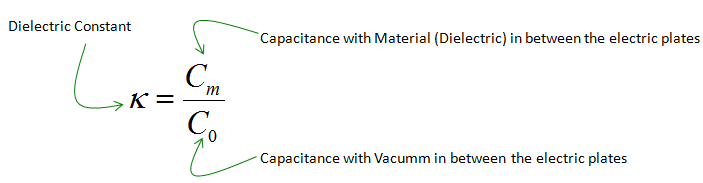Why we care about Dielectric Constant ?

If you are still not clear about the definition of the Dielectric Constant, just hold it on there and just try to understand how it affects physical characteristics that you are familiar with. As you see more and more of those examples, you would get stronger motivation to understand the definition and get back to what is described above. Then, the definition parts would come to you with more intimate face.

< Effect on Electric Force >

This example shows how Dielectric Constant influece the force between two electric charges. As you might have learned from your high school physics, the strength of Force is proportional to the multiplication of amount of two charges. and the strength of Force is in inversally protportional to the "distance squared" between the charges.

Also the force is inversally proportional to the permitivity of the material between two charges.

The illustration and equation on the left side is the case where there is no other material between the two charges. You can also say that the material between the two charges is vacume.

The illustration and equation on the right side is the case where there is a dielectric material between the two charges. As you see in the equation, the Force between charges is in inversely proportional to the permitivity of the dielectric material. It means that as the permitivity of the dielectric material goes higher, the force between charges gets smaller.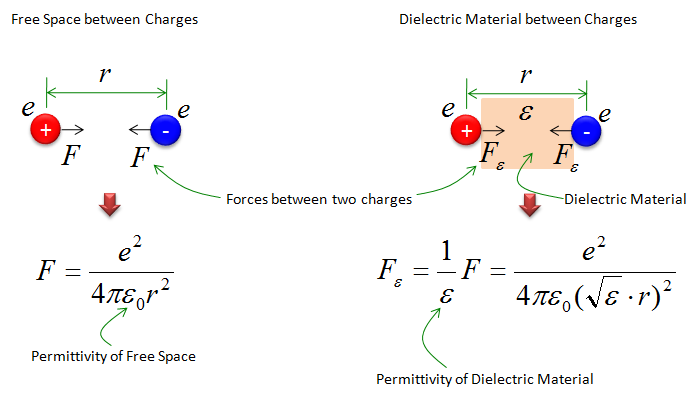< Effect on Capacitance >

Now let's look into another example. This is about the capacitance. The equation (formular) shown below tells about the comparizon of a capacitance with no material (vacume) and the one with dielectric material between two electric plates.

The formula below tells "The larger the dielectric constants of the material in the capacitor is, the larger the capacitance becomes".

For example, if you put a dielectric material with larger dielectric constant between the two plates when you make a capacitor, you would get a capacitor with larger capacitance value.< Effect on Wavelength >

If most of RF and Microwave engineers, this would be the most practical example. Following is the equation defining the wavelenth from the frequency and speed of light. The first part is the definition you are familiar from High school physics which assume that the wave propagate through vacume.

When the electromagnetic wave propagate through a diectric material, the wavelength is in inversely proportional to relative mermitivity (Dielectric constant). It means that as dielectric constant gets larger, wavelength gets shorter. It also mean that dielectric constant can influence the size of the RF/Microwave devices which are influenced by wavelength.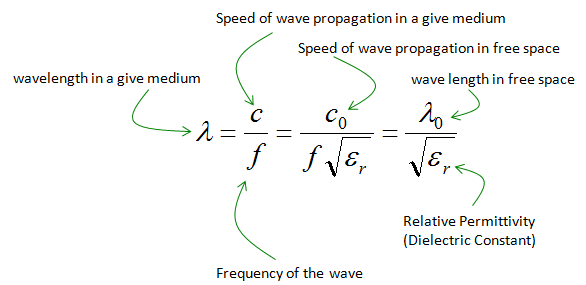Dielectric Constants for well known Materials

Following is a list of dielectri material which are widely used in electric device and RF/Microwave device manufacturing.

Material

Dielectric Constant

Vacuum

1.0

Air

1.0006

Teflon(PTFE : Polytetrafluoroethylene)

2.1

Rubber

2.8

Paper

3.0

Cocaine

3.1

Mica

3 ~ 6

Quartz

4.2

FR-4

4.8

Glass

7.8 (5~10)

Zircon

12

Ethyl Alcohol

24

Water (212 deg F)

55.3

Water (32 deg F)

88